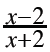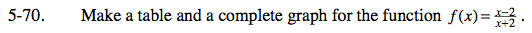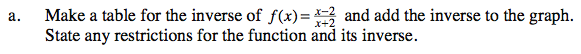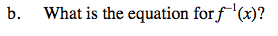### Home > INT3 > Chapter 5 > Lesson 5.2.2 > Problem5-70

5-70.
1. Make a table and a complete graph for the function f(x) =. Homework Help ✎

1. Make a table for the inverse of f(x) =and add the inverse to the graph. State any restrictions for the function and its inverse.

2. What is the equation for f –1(x)?Use integer values from −4 to 0 for x in your table.Switch the x and y coordinates in your original table.$f^{-1}\left(x\right)=\frac{-2x-2}{x-1}\text{ or }f^{-1}\left(x\right)=-\frac{4}{x-1}-2$